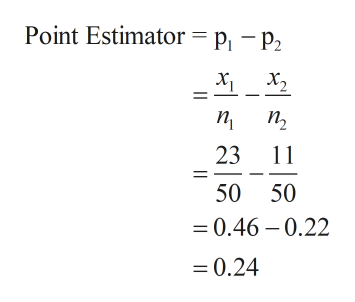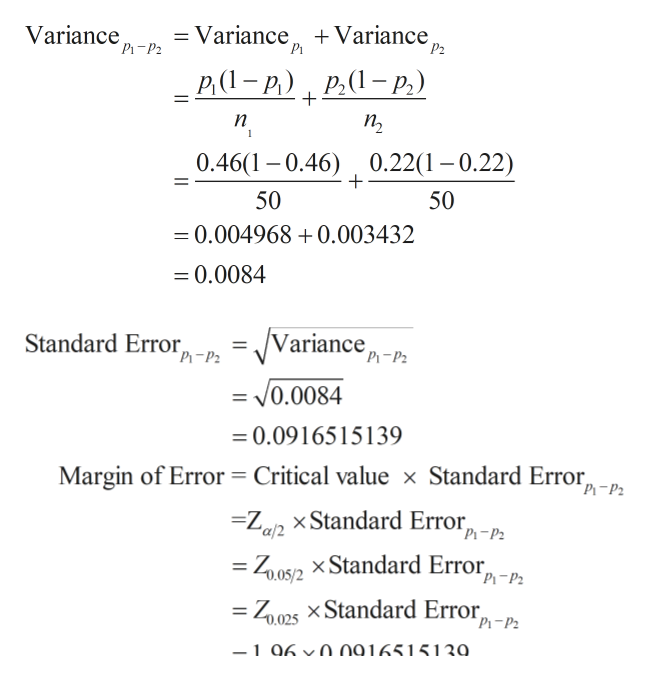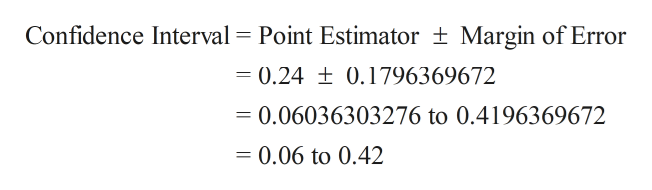# Reduction of 3+ PointsTreatment          n        Number     ProportionNew                   50       23           0.46Standard           50       11            0.22Construct a 95% confidence interval for the difference in proportions (risk difference) of patients reporting between treatments.

Question
15 views

Reduction of 3+ Points

Treatment          n        Number     Proportion

New                   50       23           0.46

Standard           50       11            0.22

Construct a 95% confidence interval for the difference in proportions (risk difference) of patients reporting between treatments.

check_circle

Step 1

Sample proportions must first be determined by taking ratios of successes to sample size. Let the sample proportions be p1 and p2.

Point estimator will be difference of the two sample proportions and can be calculated as shown below.help_outlineImage TranscriptionclosePoint Estimator= p1 - P2 п, п 23 1 50 50 0.46 0.22 -0.24 fullscreen
Step 2

p1 =0.46 and p2=0.22

First we'll calculate variance which is necessary to find standard error which is required to find margin of error which in turn is required to calculate Confidence Interval of difference of meanshelp_outlineImage TranscriptioncloseVariance Рі - Р2 + Variance = Variance P2 Р.(1- P.) Р.(1—- р) п, п 1 0.46(10.46) 0.22(1-0.22) 50 50 =0.004968 0.003432 =0.0084 = /Variance, Standard Error 'P-P2 0.0084 =0.0916515139 Margin of Error = Critical value x Standard Error, P-P2 Zg xStandard Error, "a/2 "Рі-P2 = Z05/2 XStandard Error, "P -Р. = Z025 XStandard Error, Рi -P2 -1 060 n916515130 fullscreen
Step 3

Confidence interval of difference of means can be calculated uisng point es...help_outlineImage TranscriptioncloseConfidence Interval Point Estimator Margin of Error = 0.24 ± 0.1796369672 0.06036303276 to 0.4196369672 0.06 to 0.42 fullscreen

### Want to see the full answer?

See Solution

#### Want to see this answer and more?

Solutions are written by subject experts who are available 24/7. Questions are typically answered within 1 hour.*

See Solution
*Response times may vary by subject and question.
Tagged in

### Measures